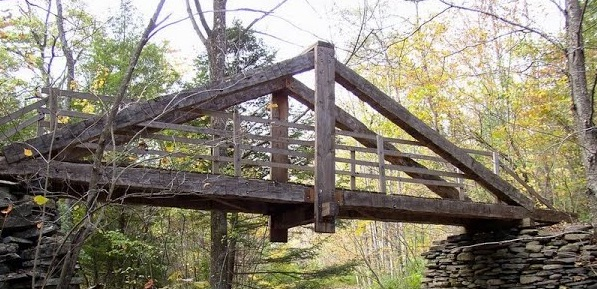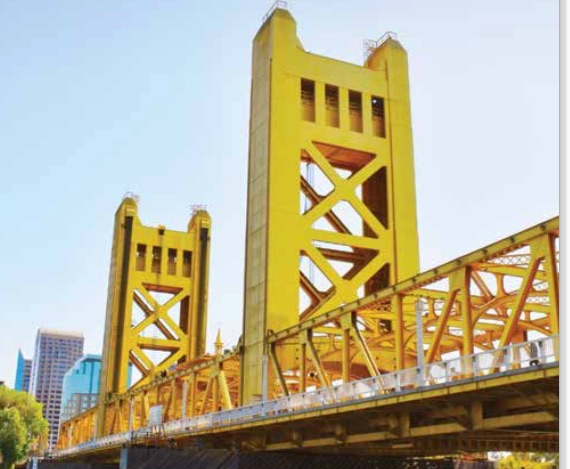# Applications of Congruent Triangles

There are many applications of congruent triangles as you saw in the videos.

For each of the following, draw a figure and explain.

1. In the first video you saw them using triangles to make the gate.   Your task is to  make a rectangular garden.   You have two  $$5’$$ boards and two $$10’$$ boards.  Draw a diagram and explain how you would use congruent triangles to make sure that the garden is rectangular.
2. There are many examples of bridges that use congruent triangles.  As in the popsicle stick bridge, equilateral triangles were used.  How could you use what you know about congruent triangles to make sure that the triangles are all the same?
3. Looking at the bridge (Math on McKenszie website), you will see the tressel,  how do you know that the right triangles are congruent in each of the supports?  How do you know that the isosceles triangles are congruent?1. The length of the bridge above is 20′ and the angles formed by the base and the hypotenuse is 45˚.   Using congruent triangles and properties of right triangles, find the lengths of the pieces of the tressel.   See the diagram below.Picture taken from California’s driver’s manual.1. How was congruent triangles used in constructing the bridge?  If we take one part of the bridge between the pillars, there are 8 rectangular pieces with a cross beam.  What lengths do you need for each piece?   We will assume that between the pillars is $$100′$$long.  and the height is $$10’$$.